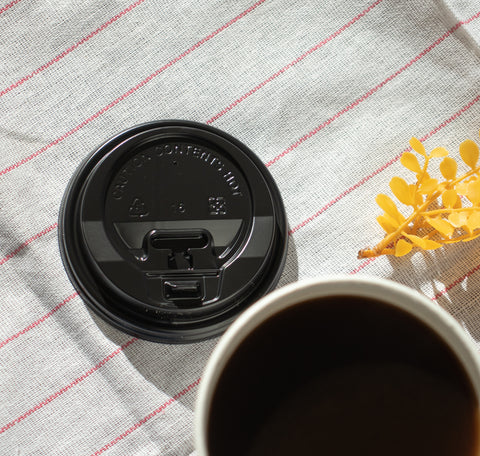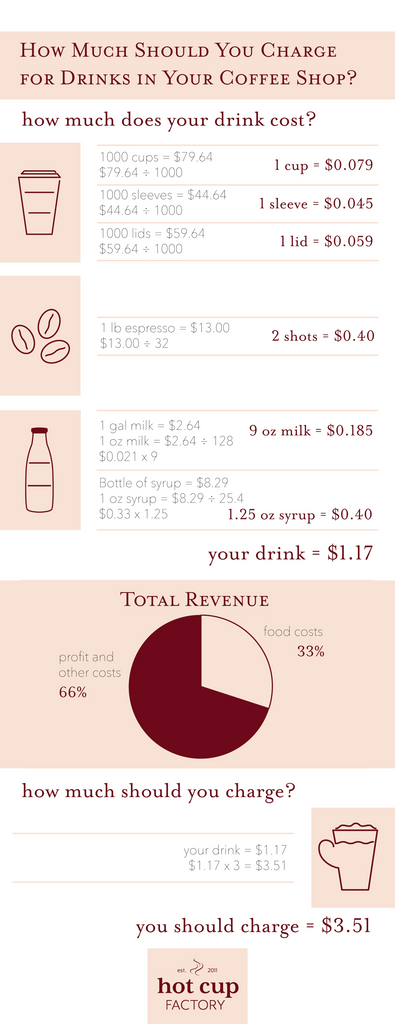# How to Calculate the Price of Drinks in Your Coffee Shop

When opening a coffee shop, creating your menu is a big task. Once you've figured out what drinks you'll be serving, you'll need to price your drinks. There's not a flat price you should charge for everything. Here's a simple guide for how to calculate the price of drinks in your coffee shop.

## Let's first consider a 16 oz latte.

### The Cup:A full case of 16 oz single wall white hot paper cups costs \$79.64. One case has 1000 cups. To calculate the price of cups, you need to divide 79.64 by 1000. This means each cup costs 7.9 cents.

### The Sleeve:

A full case of kraft cup sleeves costs \$44.64. One case has 1000 sleeves. To calculate the price of each sleeve, divide 44.64 by 1000. Each sleeve costs 4.5 cents.

### The Lid:A full case of white hot paper cup lids costs \$59.64. Each case has 1000 lids. If you divide 59.64 by 1000, you'll find that each lid costs 5.9 cents.

### The Coffee:

A 16 oz latte has 2 shots of espresso. Assuming a pound of espresso costs \$13 and there are approximately 64 shots of espresso in a pound, you'll have to divide 13 by 64. This means that each shot costs 20 cents. Because your latte has two shots, your coffee will cost 40 cents.

### The Milk:In a 16 oz latte, you'll have about 9 oz of milk in each cup. A gallon of milk costs \$2.64. There are 128 oz in a gallon. If you divide 2.64 by 128, you'll see that each ounce costs 2.1 cents. Because your latte has 9 oz of milk, you'll spend 18.5 cents for milk in each 16 oz latte.

### The Syrup:

Most lattes have 1.25 oz of syrup. One bottle of syrup costs \$8.29. Each bottle has 25.4 oz. If you divide 8.29 by 25.4, you'll see that each ounce costs 33 cents. If you multiply that by 1.25, you'll see that each latte has 40 cents of syrup.

Altogether, that's \$1.17. A successful restaurant spends 33% or a third of their revenue on food costs. This means to calculate the total price you should charge for your drinks, you should multiply the total cost of making your drinks by three. For the latte we built above, your total price will be \$3.51Calculating the cost of every menu item may take a lot of time, but it's worth the work. Charging very specific amounts for each item will allow you to keep your revenue and profit steady.